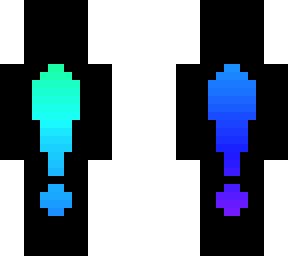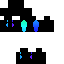### Inactivity Notice

Posted on: Jul 12, 2021
36
36
0
~=~=~=~=~=~=~=~=~=~=~=~=~=~
So you may have noticed that I'm
I'm running out of ideas, and I
just got a new computer
So I'm taking a break for a bit
Ill be back in about a month
Not quiting
~=~=~=~=~=~=~=~=~=~=~=~=~=~
shoutout to:
Ravagelag
MIIIIIIKKO
mxstymoon
SkyeCreates
yeetgamer213
Derpdoge1234
mochhi
suerskinz
itzblaze
~=~=~=~=~=~=~=~=~=~=~=~=~=~
k bye
~=~=~=~=~=~=~=~=~=~=~=~=~=~
zeep
Show Less

### Inactivity Notice

Posted on: Jul 12, 2021
36
36
0
~=~=~=~=~=~=~=~=~=~=~=~=~=~
So you may have noticed that I'm
I'm running out of ideas, and I
just got a new computer
So I'm taking a break for a bit
Ill be back in about a month
Not quiting
~=~=~=~=~=~=~=~=~=~=~=~=~=~
shoutout to:
Ravagelag
MIIIIIIKKO
mxstymoon
SkyeCreates
yeetgamer213
Derpdoge1234
mochhi
suerskinz
itzblaze
~=~=~=~=~=~=~=~=~=~=~=~=~=~
k bye
~=~=~=~=~=~=~=~=~=~=~=~=~=~
zeep